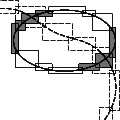CGAL 5.3 - Intersecting Sequences of dD Iso-oriented Boxes
Intersecting Sequences of dD Iso-oriented Boxes ReferenceLutz Kettner, Andreas Meyer, and Afra Zomorodian
An efficient algorithm for finding all intersecting pairs for large numbers of iso-oriented boxes, in order to apply a user defined callback on them. Typically these boxes will be bounding boxes of more complicated geometries. The algorithm is useful for (self-) intersection tests of surfaces etc.
Introduced in: CGAL 3.1
BibTeX: cgal:kmz-isiobd-21a
Windows Demo: Polyhedron demo
Common Demo Dlls: dlls

## Concepts

• BoxIntersectionBox_d
• BoxIntersectionTraits_d

## Classes

• CGAL::Box_intersection_d::Box_d<NT,int D,IdPolicy>
• CGAL::Box_intersection_d::Box_with_handle_d<NT, int D, Handle, IdPolicy>
• CGAL::Box_intersection_d::Box_traits_d<BoxHandle>

## Functions

• CGAL::box_intersection_d
• CGAL::box_self_intersection_d
• CGAL::box_intersection_all_pairs_d
• CGAL::box_self_intersection_all_pairs_d

## Modules

Concepts

Classes

CGAL::box_intersection_d()
The function box_intersection_d() computes the pairwise intersecting boxes between two sequences of iso-oriented boxes in arbitrary dimension.

CGAL::box_intersection_all_pairs_d()
The function box_intersection_all_pairs_d() computes the pairwise intersecting boxes between two sequences of iso-oriented boxes in arbitrary dimension.

CGAL::box_self_intersection_d()
The function box_self_intersection_d() computes the pairwise intersecting boxes in a sequence of iso-oriented boxes in arbitrary dimension.

CGAL::box_self_intersection_all_pairs_d()
The function box_self_intersection_all_pairs_d() computes the pairwise intersecting boxes in a sequence of iso-oriented boxes in arbitrary dimension.